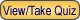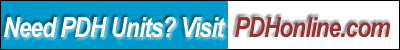Print this page

Symmetrical Components

Lee Layton, P.E.

Course Outline

This course begins with a review of the complex math involved in analyzing symmetrical components including polar and rectangular coordinates, complex number multiplication, and matrix analysis.

Next, the concept of sequence components is discussed including how to break a three-phase electric power system waveform into positive, negative, and zero sequences.  The process to convert real-time values into per-unit values is reviewed.

The various sequence networks used to analyze a system are discussed including how to model generators, transformers, and transmission lines.  Finally an example is used to bring it all together and demonstrate the application of symmetrical components to a simple engineering problem.

This course includes a multiple-choice quiz at the end, which is designed to enhance the understanding of the course materials.

Learning Objective

After taking this course you should:

• Understand the conversion from polar to rectangular coordinates;
• Know how to handle complex math concepts solve fault conditions;
• Understand the “a” operator;
• Know how to perform matrix algebra;
• Understand the components of a three-phase power waveform;
• Understand how to convert a three-phase power waveform into positive, negative, and zero sequences;
• Know how to convert real quantities to per-unit values;
• Know how to connect sequence networks for various fault types;
• Know how to model a generator, transformer, and transmission line;
• Understand the connections for three-phase faults;
• Understand the connections for line-to-ground faults; and
• Understand the proper application of symmetrical components to unbalanced system conditions.

Intended Audience

This course is intended for electrical engineers and others who want to understand how electric power systems are modeled.

Benefit to Attendees

Today, complex power system problems are analyzed used computer algorithms and it is easy to forget how the system is modeled.  This course is a review of how symmetrical components are applied to power systems and will help you to understand the basis for how the modeling behaves.

Course Introduction

The analysis of a steady-state three-phase electrical system is relatively simple to perform by using a per-phase equivalent circuit.  This method is only valid though when the voltages and currents are balanced (i.e. equal magnitudes and displaced 120 degrees apart) and when each phase has the same impedance. The analysis becomes much more difficult when the phase voltages and currents are unbalanced such as occurs during unbalanced faults.  Examples of unbalanced faults include single-line-to-ground, double-line-to-ground, and line-to-line faults.

In 1918, Charles Fortescue presented the now classic paper “Method of symmetrical component coordination applied to the solution of polyphase networks” to the American Institute of Electrical Engineers (now known as IEEE).  While his approach is useful for any number of phase conductors, this discussion is limited to three-phase networks.

The basic premise of symmetrical components is that an unbalanced network of three related vectors can be resolved into three sets of vectors.  Two of the sets have equal magnitude and are displaced 120 degrees apart while the third set has equal magnitude, but zero phase displacement.  The three sets are known as the positive, negative, and zero sequence components of the electrical system.

To study the use of symmetrical components we will first review the math that is used in solving symmetrical component equations and the application of per-unit calculations to electric power systems.  Then we will study system components in detail including component schematics and network connections.  Finally, we will use an example to bring it all together.

Course Content

This course content is in the following PDF document:

Symmetrical Components

Course Summary

The application of symmetrical components takes an unbalanced network of three related vectors and resolves them into three sets of vectors.  Two of these sets have equal magnitude and are displaced 120 degrees apart while the third set has equal magnitude, but zero phase displacement.  To use symmetrical components we must know the sequence network connections for various fault types and sequence connections for different components.

Applying the concepts of symmetrical components to three-phase electric power networks makes the analysis of unbalanced conditions manageable.  Even with symmetrical component analysis, the application can be tedious and most engineers use sophisticated computer systems to model unbalanced conditions.  It is worthwhile though, to understand how symmetrical components are used to analyze electric systems.

Quiz

Once you finish studying the above course content, you need to take a quiz to obtain the PDH credits.DISCLAIMER: The materials contained in the online course are not intended as a representation or warranty on the part of PDH Center or any other person/organization named herein. The materials are for general information only. They are not a substitute for competent professional advice. Application of this information to a specific project should be reviewed by a registered architect and/or professional engineer/surveyor. Anyone making use of the information set forth herein does so at their own risk and assumes any and all resulting liability arising therefrom.